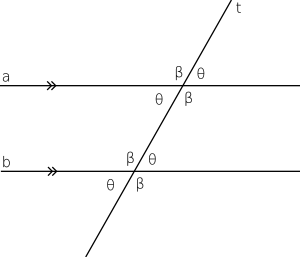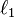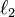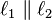# Parallel (geometry) facts for kids

Kids Encyclopedia FactsWe can see that lines a and b are parallel because the angles between the lines and an intersecting line are the same.

Parallel is a term in geometry and in everyday life that refers to a property of lines or planes. Parallel lines or planes are next to each other, but never touch each other. This means they never intersect at any point. If two lines$\ell_1$ and$\ell_2$ are parallel, then we describe this by writing$\ell_1 \parallel \ell_2$. The slopes of parallel lines are always equal.

Even if these two line segments were extended to infinity, there would never be a point of intersection between the two of them. In fact, two parallel lines in the two-dimensional plane are always a fixed distance apart.

## Related pagesParallel (geometry) Facts for Kids. Kiddle Encyclopedia.